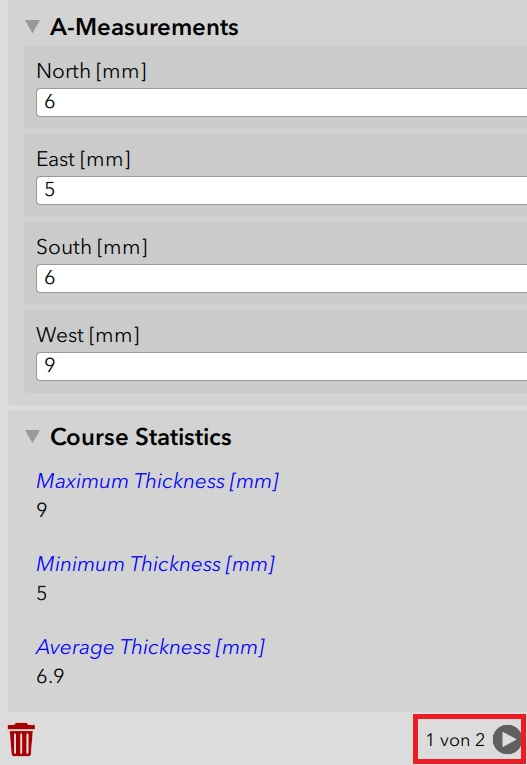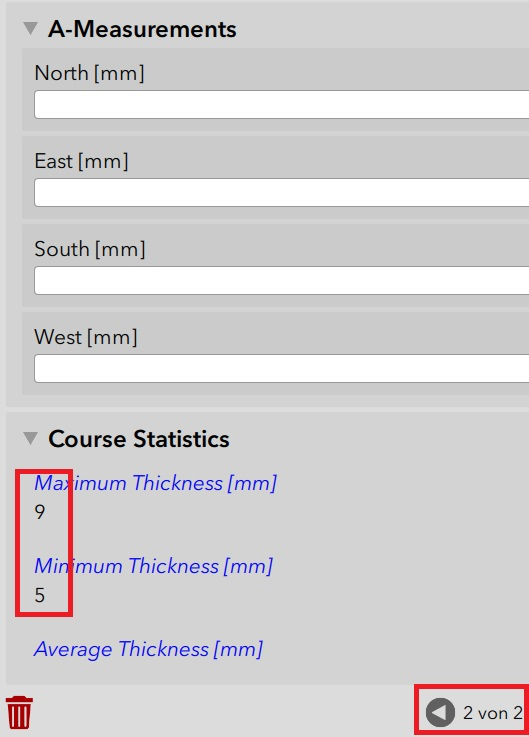# Calculations on an unknown number of fields

189
6
11-29-2018 06:18 AM

Hello,

I have a survey for a number of measurements, in total 32. The measurements compose a matrix of 4 by 8 (4 different heights, 8 different wind directions). Not all points must be measured, it is up to the field guy which measurements he/she takes.

All possible measurement points are represented by an integer question in my survey. For various reasons I am not using repeats.

Now, I want to make some calculations like max(), min(), sum(), avg(), but only for those measurement points that do have a value.

Does anyone have a tip how I can tackle this task (efficiently) in Survey123?

Thank you very much, best regards,

Jürgen

Tags (3)
6 Replies
byEsri Esteemed Contributor

Hi Jürgen,

There are a couple of ways to do this:

- if you have a few calculations, you can use the coalesce() function to provide an alternative value if a question is not answered (null) - coalesce(\${q1}, 0) will return the value in q1 when present, 0 otherwise

- an alternate formula to coalesce would be: if(string-length(\${q1}) = 0, 0, \${q1})

- If you have a large number of calculations, you can add a calculate question after each measurement to store the coalesce or if/string-length function (likely making the bind:esri:fieldType null to not add fields to the table) and use the calculate questions' values instead.

Hi James,

Thank you for replying to my question. The coalesce() function is new to me and I think it can be helpful in the future. Nevertheless, I hoped that there would be an easier way to achieve the calculation results I am looking for. Right now and with regards to what you are writing may solution would be as follows:

-> For each edited field of the 32 fields - calculate, if it is the maximum and set the maximum question

-> For each edited field of the 32 fields - calculate, if it is the maximum and set the minimum question

-> Sum up all edited fields

-> Find out how many fields have been edited

-> Calculate the average value of all edited fields

Since I can only do one calculation per question I would need a lot of calculation questions to accomplish the task.

My hope was that I can kind of tag all fields that were edited. Then, the calculations would be:

-> max(all tagged fields)

-> min(all tagged fields)

-> sum(all tagged fileds) div count (all tagged fields)

Best regards,

Jürgen

byEsri Esteemed Contributor

Hi Jürgen,

The max() and min() functions can work as you want.  The issue is sum() and count() - they are defined in the XLSForm spec to only work with repeats.

Hi James,

There seems to be a problem with the max() and min() functions in nested repeats. Please see the attached example. Here, the max() function does not work. But, as soon as you delete the outer repeat, the max() function works as expected. Any chance to get around this problem or to fix it?

As for the average function: I found a solution in an older thread here in GeoNet -> How to implement this formula in Excel Form (Survey123)

But, in my case with 32 questions to be averaged, this solution seems to be very cumbersome. Can you think of an easier way to do this? (Please also see the attached survey which has only 8 integer questions. The intended structure is the same but with four groups of eight integer questions each.)

Thank you very much, best regards,

Jürgen

byEsri Esteemed Contributor

Hi Jürgen,

Thanks for bringing this example.  Support for aggregation functions is still be examined.

Hi James,

Meanwhile I found ways to calculate min(), max(), and avg() for my fields. But now I see other problems. I have up to eight measurements for one object. For these measurements I calculate min(), max(), and avg(). The object is in a repeat, i. e. I can have more than one object. I want to calculate min(), max(), and avg() individually for each object.

1. The calculation of min(), max(), and avg() for the first object is working perfectly. But when I create the second object, min() and max() of the first object are retained (here with four measurements).2. Although I set "allowUpdates=true query=’1=1’" in column "bind::esri:parameters" for this repeat, some of the values are not loaded down to the surveys in my inbox.

I am using note questions to display the calculated values since it does not work with integer or decimal questions. Maybe these observations help to narrow down the problem with calculations in a repeat.

Best regards,

Jürgen